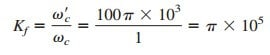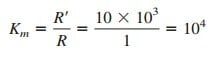# Simple Magnitude and Frequency Scaling in Filter CircuitsIn designing and analyzing filters and resonant circuits or in circuit analysis in general, it is sometimes convenient to work with element values of 1 Ω, 1 H, or 1 F, and then transform the values to realistic values by scaling. Now we will make use of Magnitude and Frequency Scaling.

Contents

We have taken advantage of this idea by not using realistic element values in most of our examples and problems; mastering circuit analysis is made easy by using convenient component values. We have thus eased calculations, knowing that we could use scaling to then make the values realistic.

There are two ways of scaling a circuit: magnitude or impedance scaling, and frequency scaling. Both are useful in scaling responses and circuit elements to values within the practical ranges. While magnitude scaling leaves the frequency response of a circuit unaltered, frequency scaling shifts the frequency response up or down the frequency spectrum.

## Magnitude Scaling

Magnitude scaling is the process of increasing all impedance in a network by a factor, the frequency response remaining unchanged.

Recall that impedances of individual elements R, L, and C are given by

In magnitude scaling, we multiply the impedance of each circuit element by a factor Km and let the frequency remain constant. This gives the new impedances as

Comparing Equation.(2) with Equation.(1), we notice the following changes in the element values: R KmR, L KmL, and C C/Km. Thus, in magnitude scaling, the new values of the elements and frequency are

The primed variables are the new values and the unprimed variables are the old values. Consider the series or parallel RLC circuit. We now have

showing that the resonant frequency, as expected, has not changed. Similarly, the quality factor and the bandwidth are not affected by magnitude scaling.

Also, magnitude scaling does not affect transfer functions in the previous post in ‘Learn What is Transfer Function Quickly’, which are dimensionless quantities.

## Frequency Scaling

Frequency scaling is the process of shifting the frequency response of a network up or down the frequency axis while leaving the impedance the same.

We achieve frequency scaling by multiplying the frequency by a factor
Kf while keeping the impedance the same.

From Equation.(1), we see that the impedances of L and C are frequency-dependent. If we apply frequency scaling to ZL(ω) and ZC(ω)
in Equation.(1), we obtain

since the impedance of the inductor and capacitor must remain the same
after frequency scaling. We notice the following changes in the element
values:
L L/Kf and C C/Kf . The value of R is not affected, since its impedance does not depend on frequency.

Thus, in frequency scaling, the new values of the elements and frequency are

Again, if we consider the series or parallel RLC circuit, for the resonant
frequency

and for the bandwidth

but the quality factor remains the same (Q’ = Q).

## Magnitude and Frequency Scaling

If a circuit is scaled in magnitude and frequency at the same time, then

These are more general formulas than those in Equations.(3) and (6). We set Km = 1 in Equation.(9) when there is no magnitude scaling or Kf = 1 when there is no frequency scaling.

## Magnitude and Frequency Scaling Example

A fourth-order Butterworth lowpass filter is shown in Figure.(1a). The filter is designed such that the cutoff frequency ωc = 1 rad/s. Scale the circuit for a cutoff frequency of 50 kHz using 10-kΩ resistors.Also, if each 1-Ω resistor is to be replaced by a 10-kΩ resistor, then theThe scaled circuit is as shown in Figure.(1b). This circuit uses practical